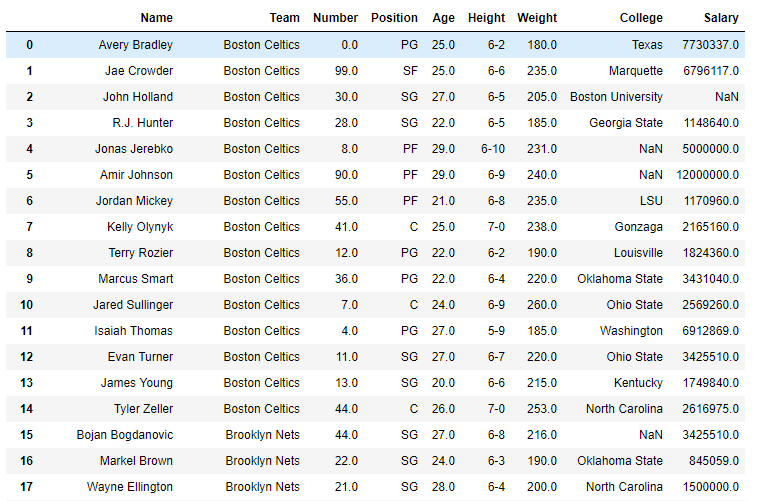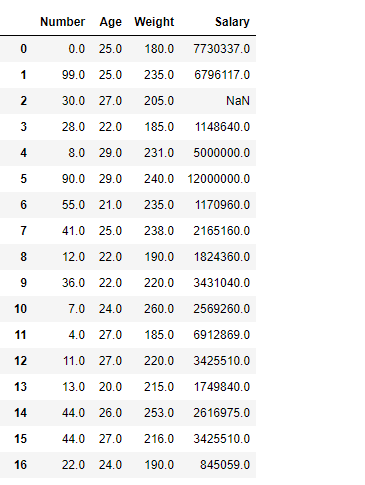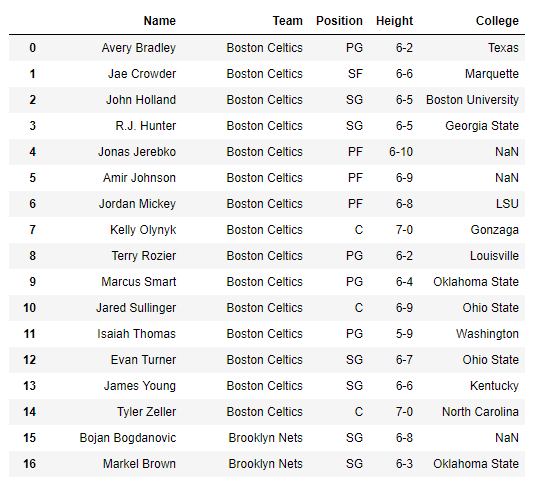Open In App

# Python | Pandas dataframe.select_dtypes()

Python is a great language for doing data analysis, primarily because of the fantastic ecosystem of data-centric python packages. Pandas is one of those packages and makes importing and analyzing data much easier.

Pandas` dataframe.select_dtypes()` function return a subset of the DataFrame’s columns based on the column dtypes. The parameters of this function can be set to include all the columns having some specific data type or it could be set to exclude all those columns which has some specific data types.

Syntax : DataFrame.select_dtypes(include=None, exclude=None)

Parameters :
include, exclude : A selection of dtypes or strings to be included/excluded. At least one of these parameters must be supplied.

Return : The subset of the frame including the dtypes in include and excluding the dtypes in exclude.

Example #1: Use `select_dtypes()` function to select all the columns which are having floating data types.

 `# importing pandas as pd``import` `pandas as pd`` ` `# Creating the dataframe ``df ``=` `pd.read_csv(``"nba.csv"``)`` ` `# Print the dataframe``df`Let’s use the `dataframe.select_dtypes()` function to select all columns having float data type in the dataframe.

 `# select all columns having float datatype``df.select_dtypes(include ``=``'float64'``)`

Output :Example #2: Use `select_dtypes()` function to select all the columns in the dataframe except those columns which are of float data type.

 `# importing pandas as pd``import` `pandas as pd`` ` `# Creating the dataframe ``df ``=` `pd.read_csv(``"nba.csv"``)`` ` `# select all columns except float based``df.select_dtypes(exclude ``=``'float64'``)`

Output :